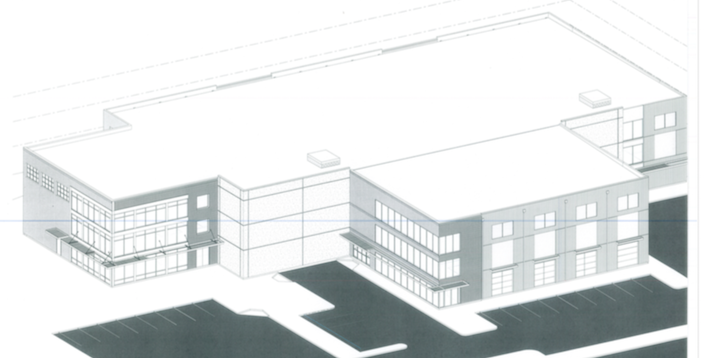# Cap Rate

#### What is Capitalization Rate (Cap Rate)?

Capitalization rate (or “Cap Rate”) is a real estate valuation measure used to compare different real estate investments. Although there are many variations, a cap rate is often calculated as the ratio between the net operating income (NOI) produced by an asset and the original capital cost (the price paid to buy the asset) or alternatively its current market value.

## Basic formula is calculated as follows:

CAP RATE = NET OPERATING INCOME /ASSET  VALUE

## For Example:

If an apartment is purchased at the sale price of \$6,000,000 and the net operating income (NOI) produced in one year is \$600,000…, then:

##### \$600,000 / \$6,000,000 = 0.10 = 10%

The asset’s cap rate is ten percent; one-tenth of the building’s cost is paid by the year’s net proceeds.

Investors can also use this basic formula to determine if the valuation of an apartment or asset asking price is undervalued or overvalued in terms of market price.

## Another Example:

The same apartment is on the market for \$10,000,000 and has an NOI of \$600,000. Use the following formula to calculate its cap rate.

##### \$10,000,000/\$600,000 = .06 or 6% cap rate

If market comps are selling at a 10% cap rate then the above asset could potentially be priced above market values.  If the cap rate in the market are selling at 5% then this asset may be deemed undervalued and can potentially represent a good value for an investor.While determining a property’s cap rate is a great tool to help you value a property, it is not the only calculation that you should use.  There are many factors can calculations that come into play such as Debt Yield Ratio, Cash-on-Cash Return, Debt Service Ratio, and Cash Flow to determine if an asset is a good investment.